# FAQ’s on Instantaneous Centre of Rotation

We know that the motion of a wheel is a combination of translational as well as rotational motion. So instantaneous centre of rotation (ICOR) is the point from where the motion can be analyzed as a pure rotational motion and it is an important concept in rotational dynamics from JEE exam point of view. We will discuss this topic further with the help of FAQs.

## Instantaneous Axis of Rotation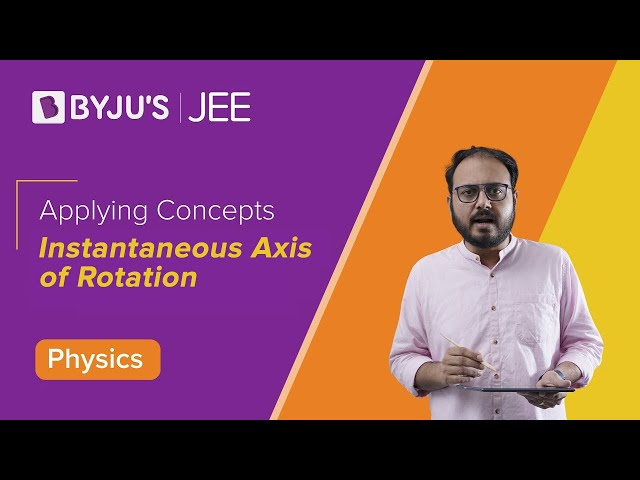## Important Questions For Instantaneous Centre of Rotation

• Discuss the concept of ICOR.

Ans – Let’s take an example of a wheel. If we assume that the wheel is in pure rolling then the lowermost point has zero velocity. So if we analyze the motion from that point at that instant then the wheel will have a pure rotational motion about that point.

• How can we locate the instantaneous centre of rotation?

Ans – We can have following different situations:

Case I : When two arbitrary velocities are given: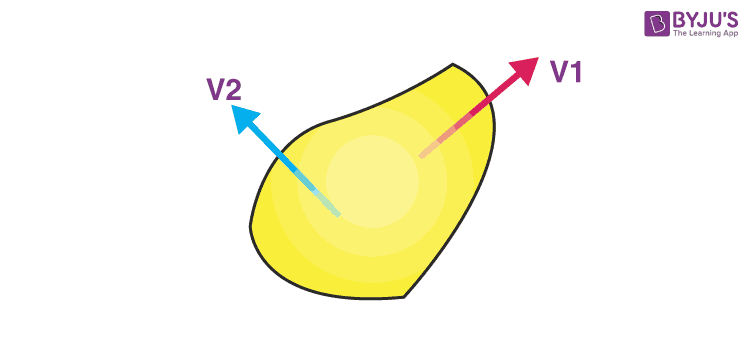We will draw a perpendicular from the two velocities, the point where they intersect is the ICOR.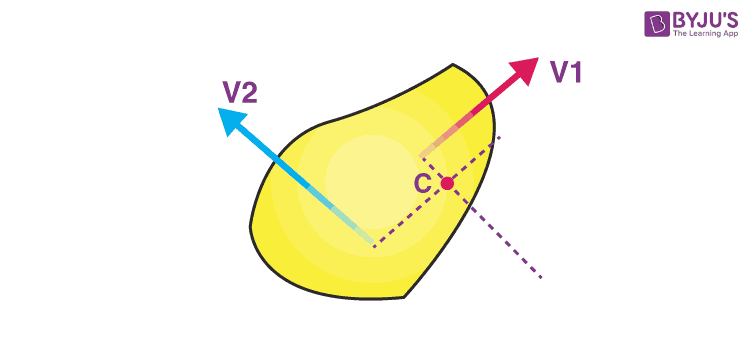Case II: When two Parallel velocities are given as shown: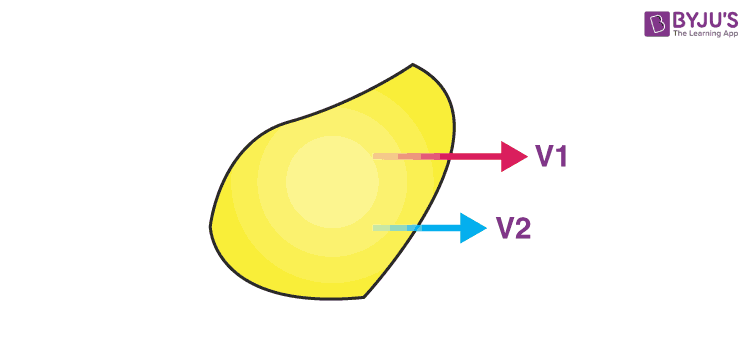Draw two perpendicular lines from the tails of the velocity vectors. Now draw a line joining their heads which intersects the perpendicular line. That point of intersection is the ICOR.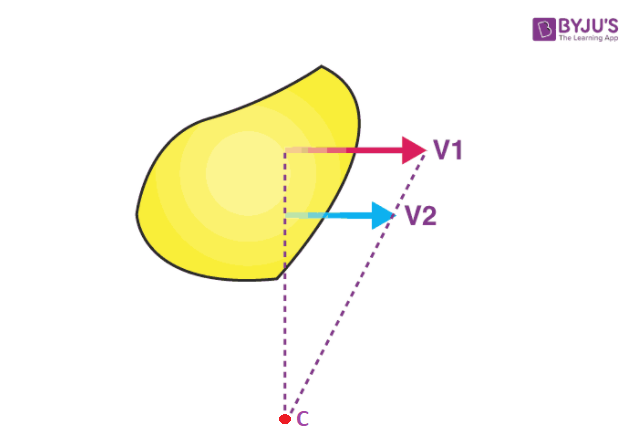Here point C is the Instantaneous centre of rotation.

• Illustrate: the importance of ICOR?

Ans – Let’s take a rod that is kept on a frictionless floor. A slight impulse is given such that it starts rotating while falling down. We have to find the velocity of the centre of mass.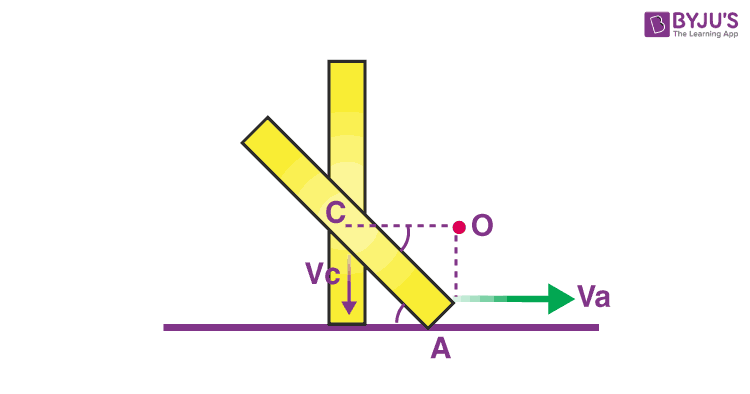Since there is no frictional force hence there is no force in the horizontal direction, so the centre of mass will only have a vertical velocity say $V_c$. Also, the lowermost point will only have a horizontal velocity. Let it be $V_a$. First, we will find the ICOR drawing lines perpendicular to the velocity vectors. They intersect at $O$. So the whole body will seem to be in the pure rotation from that point. Let the angular velocity be $V_a$. Now the centre of mass has come down by $\frac{1}{2}(1 – sin \theta)$.

By energy conservation,

$mg \frac{1}{2}(1-sin \theta) = \frac{1}{2}I_{ICOR}\ \omega^2$——- (1)

By parallel axis theorem,

$I_{ICOR} = \frac{ml^{2}}{12} = \frac{ml^{2}cos^{2} \theta}{4}$——- (2)

Using (1) and (2), we can find the value of $\omega$.

Once it is known we can find,
$V_{c}~= \omega \times \frac{1}{2} cos\theta$

Solving this equation from the ground frame can be a little complex. So this concept can be used efficiently in rotation.

To learn more tricks in order to crack JEE get in touch with our mentors here at BYJU’S.